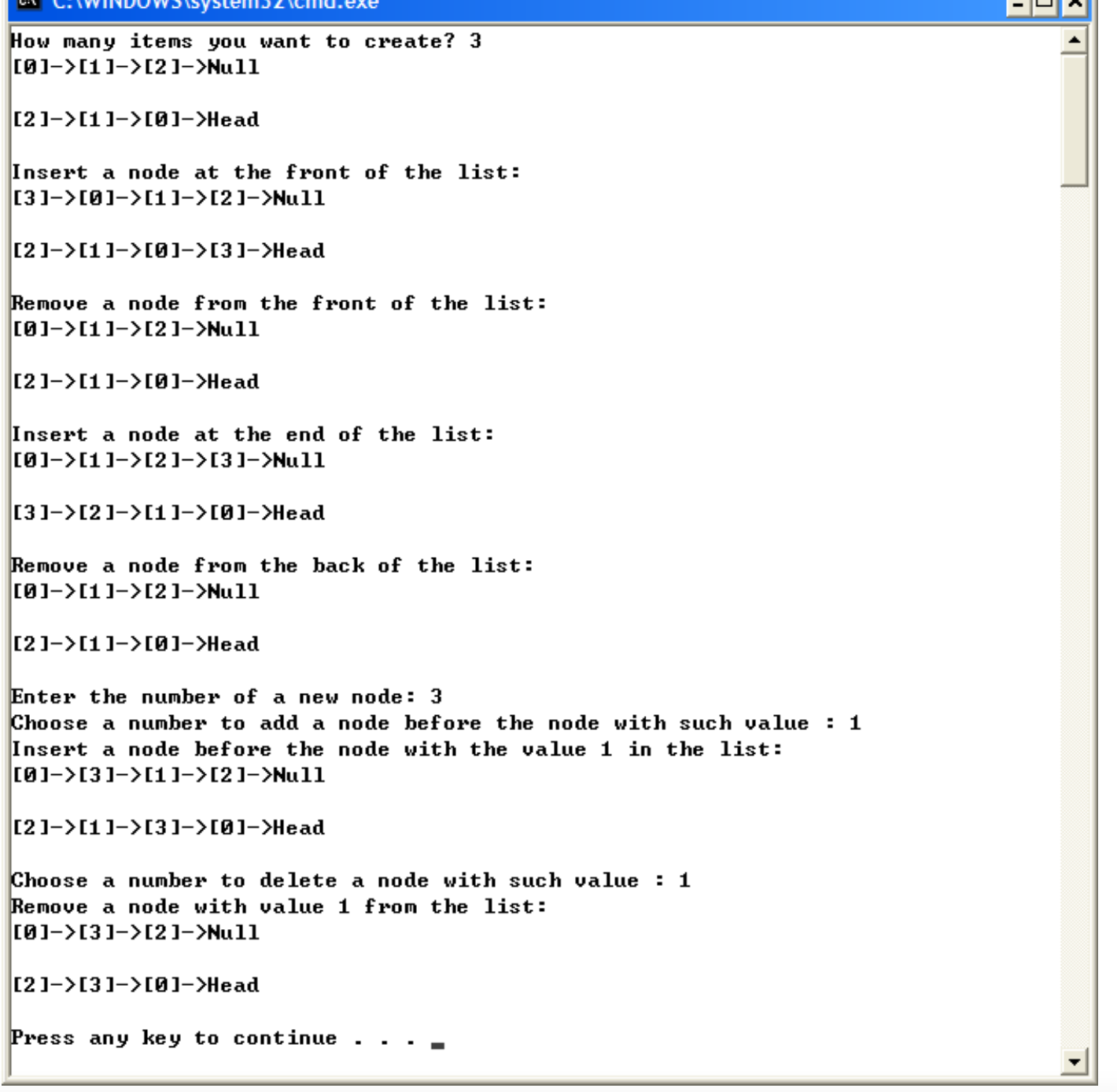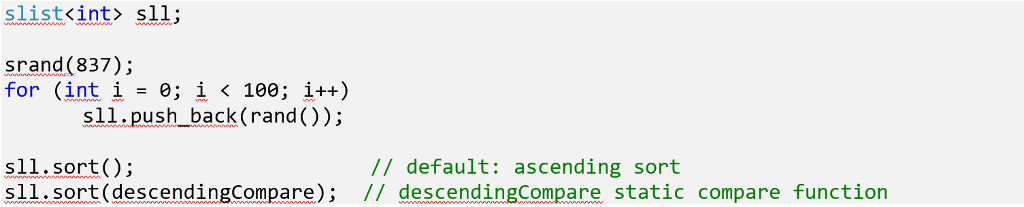Question

You are required to write the following functions using this class:

```

{

public:
// constructor initialize the nextPtr
{

prevPtr = 0; // point to null at the beginning

nextPtr = 0; // point to null at the beginning
}

// get a number
int GetNum()
{

return number;
}

// set a number
void SetNum(int num)
{

number = num;
}

// get the prev pointer
{

return prevPtr;
}

// set the prev pointer
{

prevPtr = ptr;
}

// get the next pointer
{

return nextPtr;
}

// set the next pointer
{

nextPtr = ptr;
}

private:
int number;

};

```

1 Create a doubly linked list. The integer assigned in a node of the list is its sequence (i.e., 0 is assigned in the first node, 1 is assigned in the second node, and so on.). Remember to assign the previous and next pointers properly.

2 Print a list.

3 Print a list reversely.

4 Insert a node at the front of a list

5 Remove a node from the front of a list

Insert a node at the back of a list

Remove a node from the back of a list

Insert a node before the node with a given value in a list

Remove a node with a give value in a list

You should use the following main program to test the above nine functions and do NOT modify the main program:

```
int main()
{

int num;
cout << "How many items you want to create? ";
cin >> num;
newPtr->SetNum(num);
cout << "Insert a node at the front of the list:" << endl;
cout << "Remove a node from the front of the list:" << endl;
newPtr->SetNum(num);
cout << "Insert a node at the end of the list:" << endl;
cout << "Remove a node from the back of the list:" << endl;
cout << "Enter the number of a new node: ";
cin >> num;
newPtr->SetNum(num);
cout << "Choose a number to add a node before the node with such value : ";
cin >> num;
cout << "Insert a node before the node with the value " << num << " in the list:"

<< endl;
cout << "Choose a number to delete a node with such value : ";
cin >> num;
cout << "Remove a node with value " << num << " from the list:" << endl;

return 0;
}

```

The sample output should be:Help me make these 9 c++ functions asap, keep in mind the language should be a beginners level and dont make it complicated so it allows me to understand as a beginner.

We need at least 10 more requests to produce the answer.

0 / 10 have requested this problem solution

The more requests, the faster the answer.

All students who have requested the answer will be notified once they are available.

#### Earn Coins

Coins can be redeemed for fabulous gifts.

Similar Homework Help Questions
• ### Q) Modify the class Linked List below to make it a Doubly Linked List. Name your...

Q) Modify the class Linked List below to make it a Doubly Linked List. Name your class DoublyLinkedList. Add a method addEnd to add an integer at the end of the list and a method displayInReverse to print the list backwards. void addEnd(int x): create this method to add x to the end of the list. void displayInReverse(): create this method to display the list elements from the last item to the first one. Create a main() function to test...

• ### Dynamic Lists: Pointer Issues

I have been working in Visual C++ 2008 &puTTY to figure out how to make this work. puTTY keeps giving me segmentation errors; Visual C++ gives me thefollowing:"Unhandled exception at 0x10296af2 in ForProj3.exe: 0xC0000005: Access violation writing location 0x00000000."(Note: select 5 at the switch statement)Here is where Visual C++ brings me to (lines 177-179, I tried to flag in the code text below; extra comments were removed):fscanf(dataPtr, "%d",aCtr);printf("%d read from filen",aCtr);AddToList( &sPtr, aCtr );What is causing the segmentation error? I'm...

• ### Please answer in C++ Derive a class called Queue from the linked list described in Assignment...

Please answer in C++ Derive a class called Queue from the linked list described in Assignment 2 (list of Dates). This means the Queue class will inherit all the properties (data and functions) of the linked list. But, since a queue allows pushing only at the back and popping at the front of the list, you will need to prevent the addition in the front and removal at the back. To do this, you must derive the Queue class in...

• ### C++ Create a class that implements a sorted, doubly-linked list: Start with a copy of the...

C++ Create a class that implements a sorted, doubly-linked list: Start with a copy of the sortedList class. Call your new class doublyLinkedList. Convert the baseline code into a doubly linkedlist, and thoroughly test all existing operations (make sure to check all edge conditions), and then implement the new operations below. The class should have the following additional class methods: • A reverse method: this method will reverse the order of the doubly linked list. This method takes no parameters,...

• ### Doubly Linked List The assignment is to modify the below code in any way (like changing the method of a function). Time...

Doubly Linked List The assignment is to modify the below code in any way (like changing the method of a function). Time complexity is omitted. Any methods/functions below could be changed into something different. I was thinking of changing the method of getting size of list and maybe change from numbers to letters for nodes. import java.util.Scanner; /* Class Node */ class Node { protected int data; protected Node next, prev; /* Constructor */ public Node() { next = null;...

• ### In this assignment, you will implement a sort method on singly-linked and doubly-linked lists. Implement the...In this assignment, you will implement a sort method on singly-linked and doubly-linked lists. Implement the following sort member function on a singly-linked list: void sort(bool(*comp)(const T &, const T &) = defaultCompare); Implement the following sort member function on a doubly-linked list: void sort(bool(*comp)(const T &, const T &) = defaultCompare); The sort(…) methods take as a parameter a comparator function, having a default assignment of defaultCompare, a static function defined as follows: template <typename T> static bool defaultCompare(const...

• ### Linkedlist implementation in C++ The below code I have written is almost done, I only need...

Linkedlist implementation in C++ The below code I have written is almost done, I only need help to write the definition for delete_last() functio​n. ​ Language C++ // LinkedList.cpp : Defines the entry point for the console application. // #include "stdafx.h" #include <string> #include <iostream> using namespace std; struct Node { int dataItem;//Our link list stores integers Node *next;//this is a Node pointer that will be areference to next node in the list }; class LinkedList { private: Node *first;...

• ### c++ singly linked list +

Please Help! I need to implement the following. I've written the header file, I need help with the implementation file (word.cpp) . Below are the directions:-Implement the WORD ADT (abstract data type) using a singly-linked list of characters. The WORD ADT is defined below. Call the class you implement "WORD". Define aclass called "character" that has two fields, a char field called "symbol" and a pointer field called "next". Each node (character) in the list will contain one symbolof a...

• ### You need to complete a function called insertList. Given list A, list B and an integer...

You need to complete a function called insertList. Given list A, list B and an integer n, insertList inserts list B into the middle of list A, after the list value n. NOTE: 1) All positions and indices start from 0. 2) Write your code in the empty function of insertList, DO NOT change anything in the main. Example Assume we are starting with two lists: List A is: 2->3->9->11 List B is: 5->6->8 Insert after node value: 3 Resulting...